# An Investigation into the Effect of Adding Carbon and Glass Fibres to UHMWPE Fibres on the Mechanical Characteristics of a Sports Prosthetic Foot

An Investigation into the Effect of Adding Carbon and Glass Fibres to UHMWPE Fibres on the Mechanical Characteristics of a Sports Prosthetic Foot
Mechanical Engineering Department, University of Basrah, Basrah 10066, Iraq

Materials Engineering Department, University of Technology, Baghdad 61004, Iraq

Corresponding Author Email:
hayder.talla@yahoo.com
Page:
69-76
|
DOI:
https://doi.org/10.18280/rcma.320203
10 November 2021
|
Accepted:
7 January 2022
|
Published:
30 April 2022
| Citation

OPEN ACCESS

Abstract:

Due to their qualities and advantages, such as light weight, high rigidity, and high performance, composite materials have been used in a wide variety of industries and sectors. For example, carbon fibres are used in the construction of aircraft, while ultrahigh-molecular-weight polyethylene (UHMWPE) is used in the fabrication of medical artificial joints. In this study, the blade dimensions were estimated using side profiles from a European patent specification and the mechanical properties of numerous layers of composite materials (UHMWPE, carbon, glass fibre, and Perlon) utilized in the fabrication of sports prosthesis were investigated experimentally, theoretically, and numerically, and the results were compared, as well as the theory of failure calculated. The influence of data entered into the ANSYS programme was also investigated in the case of isotropic or orthotropic materials. The findings indicate that longitudinal young modules are experimentally and theoretically equivalent. While the material ISO or Ortho is considered and its information is entered into the ANSYS programme for the same lamina, similar results are obtained under the same boundary condition, as was demonstrated when computing the theory of failure. Additionally, it was demonstrated in this research that layering woven carbon fibre on top of layers of UHMWPE woven fabrics had a greater effect than layering woven glass fibre when fabricating the sports prosthetic foot.

Keywords:

athletic prosthetic, UHMWPE, ANSYS program, carbon fiber, glass fiber

1. Introduction

Fabrics are constructed of strands that are aligned in two perpendicular directions: one is called the warp, and the other is called the fill (or weft) . The fill yarns pass between the warp yarns in a predictable order, indicating that the fibres are woven together as shown in Figure 1 . There are many forms of woven fabrics, as shown in Figure 2.

According to Bragaru et al.  individuals who have had limbs amputated may face unfavourable consequences for their psychological and physical well-being, mobility, and social lives. Participating in sports and/or engaging in regular physical activity benefits the aforementioned areas. Typically, physical appearance, mechanical behaviour, and functionality are utilised to differentiate prosthetic foot-ankle components. Traditional (Solid Ankle Cushion Heel), single-axis, multi-axis, and energy storage and return are the four fundamental foot types that have evolved and are widely used in the clinical setting . The Flex-Foot was the first prosthetic foot to have a distinctive J-shape design and to utilise carbon fibre materials .

Nolan  examined carbon fibre prosthetic feet and demonstrated that the present efficiency of the artificial foot is not equal to that of a typical natural foot. Rahman et al.  examined the performance of prosthetic running feet composed of composite material, which provide several advantages over metal counterparts, including increased weight and the ability to store a significant amount of strain energy.

## 1.png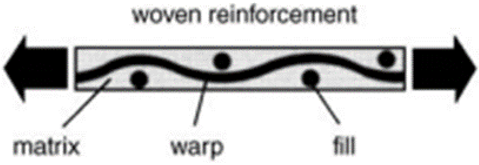Figure 1. Cross Section of woven fabrics 

## 2.png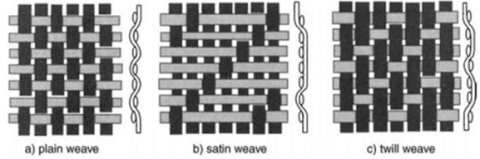Figure 2. Forms of woven fabrics 

Shasmin et al.  investigated the influence of bamboo components in prosthetic legs and found no significant difference in gait parameters. According to LeMoyne , the objective has shifted to developing prostheses capable of imparting energy during the stance phase of gait, either by energy-absorbing elasticity or powered actuation. Nielsen et al.  discovered that ambulation with the Flex-Foot was faster than with conventional prosthetic feet. Patarata  designed and constructed three distinct types of monolimb prosthesis using structural analogies to human legs. The authors analysed the stress fields using FEM and RPIM and discovered that the meshless technique generates more precise and smooth stress fields than low order finite elements. Sun and Voglewede  developed a novel powered transtibial prosthesis and demonstrated that the proposed model responds precisely, swiftly, and with redundant contact information. Kumar et al.  revealed that myoelectric prosthetic limbs require mechanical moving components, electrical circuits, bioelectronics, and material science.

The prosthetic running foot, often known as the blade, was analysed using a finite element analysis technique. According to Hawkins , the maximum foot deflection is the most realistic of the other elements to consider when selecting an athletic prosthetic. Rigney et al.  used finite element analysis to investigate the mechanical properties of two types of athletic artificial feet and discovered that the energy stored in the foot is inversely proportional to the stiffness. When doing numerical simulations of composite materials using the ANSYS workbench 16.2, Bhagavathiyappan et al.  discovered that metals with a low strain energy value are more resistant to impact loads.

Alizadeh  replaced Aluminum 7068 with composite material while maintaining the same thickness. This resulted in a weight reduction of 0.647 Kg, which, even with the additional weight of the socket cap, bolts, and shock absorber elastomer add-ons, should keep the total weight below 1Kg, which is the ideal target.

Efforts are required to close the gap in the field of prosthetic limb manufacture and to close the technology gap. Specifically, spatial investigations, experimental studies, and comparisons to numerical studies. Therefore, the mechanical properties of many layers of composite materials (UHMWPE, carbon, glass fibre, and Perlon) used in the manufacturing of sports prosthesis were investigated experimentally, theoretically, and numerically, and the results compared. The current article is divided into four sections: materials and methods, data reduction, finite element modelling, and results and discussion.

2. Materials and Methods

2.1 Materials chosen

Ultrahigh-molecular-weight polyethylene (UHMWPE) woven fabrics were bought from Yixing Huaheng High-Performance Fiber Textile Co. Ltd. in China with Perlon stockinet white. A number of layers of woven carbon fiber, woven glass fiber, and woven perlon are also added, and their mechanical properties are presented in Table 1.

The matrix used in this study was epoxy resin orthocryl lamination 80:20 pro and hardening powder (Ottobock healthcare 617P37). Table 2 shows the mechanical properties of the matrix. Figure 3 shows the woven fibers and matrix that were used in this research. The value of the share modulus was calculated from Eq. (1) .

$G_{f, m}=\frac{E_{f, m}}{2\left(1+v_{f, m}\right)}$      (1)

where: Gf, m share modulus of the fiber and matrix, respectively (GPa), Ef,m modulus of elasticity of the fiber and matrix, respectively( GPa) and v f,m Poisson’s ratio of the fiber and matrix.

Table 1. Mechanical properties of composite fibers 

 Types of fiber Ef (GPa) Gf (GPa) $\mathbf{v}_{\mathbf{f}}$ ρ (Kg/cm3) UHMWPE 114.86 39.33 0.4 0.939 carbon fiber 230 95.83 0.2 1.4 glass fiber 72.5 29.472 0.23 2.48 Perlon 3.43 1.23 0.39 1.083

Table 2. Mechanical properties of the Matrix

 Em (GPa) Gm (GPa) $\mathbf{v}_{\mathbf{m}}$ ρ (Kg/cm3) Tensile Strength (MPa) 2.5 0.829 0.38 1.19 53.8

## 3.png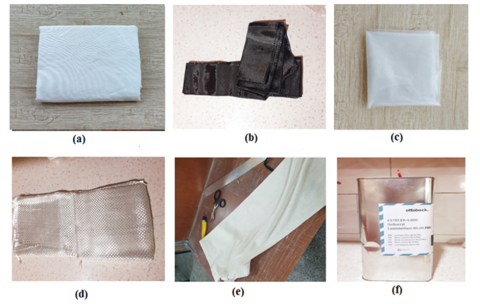Figure 3. Materials employed in the current study: (a) UHMWPE woven fabrics, (b) Woven carbon fiber, (c) Polyvinyl alcohol (PVA), (d) Woven glass fiber, (e) Woven Perlon fibers, and (f) Epoxy resin orthocryl lamination

Table 3. Types of laminations used in this study for composite material specimens

 Composite lamination system Materials Types (matrix and reinforcement) Layers' symbol Laminate 1 PMMA+UHMWPE+Perlon fibers 4p+4U Laminate 2 4p+6U Laminate 3 4p+8U Laminate4 PMMA+UHMWPE+Carbon fibers+Perlon fibers 4p+4U+2C Laminate 5 4p+6U+2C Laminate 6 4p+8U+2C Laminate 7 PMMA+UHMWPE+Glass fibers+Perlon fibers 4p+4U+2G Laminate 8 4p+6U+2G Laminate 9 4p+8U+2G

## 4.png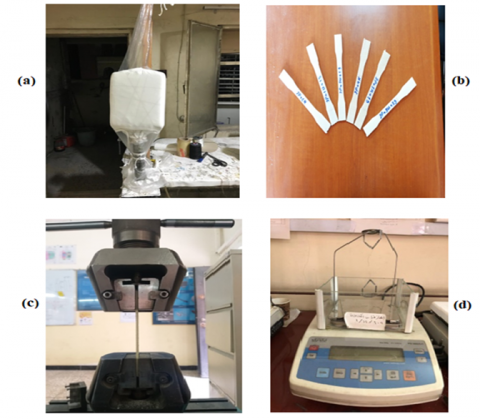Figure 4. Experimental process: (a) Vacuum bag process, (b) Tensile test specimens, (c) Tensile test process, and (d) Density tester

2.2 Composite material production

The fibers are cut into the required dimensions and weighed with an accurate scale. The models for this study were created using a two-stage manufacturing method, the first of which was hand impregnation (HAND LAY-UP), which ensures that all fibres are covered with epoxy resin when adding different layers of fibers. The second stage was the vacuum bag, in which the resin-impregnated fibres were placed on a rectangular positive Jepson mould with dimensions of 10 x 35 x 27 cm3 within a vacuum bag to achieve a homogeneous matrix surface and so extract the surplus resin by using the suction pump designated for this process, which is connected by plastic tubes with the gypsum mold, and the operation was done at room temperature as shown in Figure 4.a. The mould is then left to dry for 48 hours at an ambient temperature. After the process was repeated, eight models were manufactured with different layers, as shown in Table 3. After that, the sample is cut to the required dimensions by a CNC machine to make five tensile test specimens for each laminate for tensile testing and specimens to calculate the density of the composite material. Where the specimens of tensile tests were conducted according to ASTM D 638 as shown in Figure 4.b . The density of composite material measured is by using the density tester Radwag PS 360/C1 Precision Lab Balance, Compact Scale 360g x 0.001g,1800x0.005ct as shown in Figure 4.d according to ASTM Standards (D 792) where the density is important to calculate the volume fraction of fibre and matrix .

2.3 Mechanical testing

Mechanical testing was carried out on an Instron 3369 universal test machine with a 10 kN load cell. Tensile testing was performed in accordance with ASTM 638 using a 1 mm/min crosshead rate (Figure 4 c). This testing was used to determine the young modulus (E), ultimate tensile stress $\left(\sigma_{x}^{T}\right)_{u l t}$, and ultimate tensile strain ($\epsilon_{u}$).

3. Data Reduction

3.1 Determination of fiber volume fraction (Vf)

Fibbers are scattered throughout the matrix in a pattern that might be described as repetitive or periodic in the fibre reinforced material. Although there is some unpredictability, the cross section could be idealized as a square packed array or a hexagonal packed array as a first approximation, as shown in Figure 5 . It also shows the direction of the mechanical properties of the composite materials in the theoretical part. Because fibre and resin content have an effect on a material's mechanical reaction and quality, they should be measured and taken into account when estimating mechanical response. Experiments can be used to determine the fibre volume fraction Vf by weighing the fibres before the manufacturing process and then weighing the laminate. And using the density of the fibres mentioned in Table 3 with the density of the composite material obtained from ASTM Standards (D 792), substitute it in the Eq. (2) .

$V_{\mathrm{f}}=\frac{m_{\text {fiber}} \quad / \rho_{\text {fiber }}}{m_{\text {composite}} \quad \quad / \rho_{\text {composite}}}\cdot 100(\%)$          (2)

## 5.png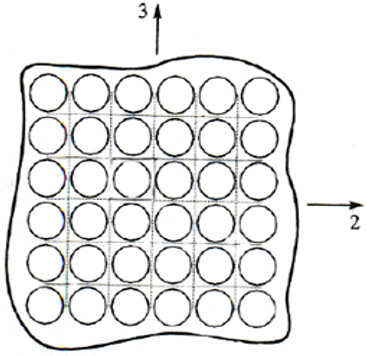Figure 5. Composite material 

3.2 Determination of the elastic characteristics

This work uses the rule of mixture to determine the properties of orthotropic composite materials. The elastic constants of woven fabric composite materials are calculated numerically by applying relationships based on constituent properties. The fill and warp directions' Young's modulus and Poisson's ratio are calculated and averaged from the longitudinal and transverse values of the respective unidirectional layer. The unidirectional composite's elastic constants are computed using the simple rule of mixtures as shown below:

$E_{1}=E_{f} V_{f}+E_{m}\left(1-V_{f}\right)$          (3)

$E_{2}=E_{m}\left[\frac{E_{f}+E_{m}+\left(E_{f}-E_{m}\right) V_{f}}{E_{f}+E_{m}-\left(E_{f}-E_{m}\right) V_{f}}\right]$            (4)

$v_{12}=v_{f} V_{f}+v_{m}\left(1-V_{f}\right)$             (5)

$v_{23}=v_{f} V_{f}+v_{m}\left(1-V_{f}\right)\left[\frac{1+v_{m}-\frac{v_{12} E_{m}}{E_{11}}}{1-v_{m}^{2}+\frac{v_{m} v_{12} E_{m}}{E_{11}}}\right]$                       (6)

$G_{12}=G_{m}\left[\frac{G_{f}+G_{m}+\left(G_{f}-G_{m}\right) V_{f}}{G_{f}+G_{m}-\left(G_{f}-G_{m}\right) V_{f}}\right]$                              (7)

$G_{23}=\frac{E_{22}}{2\left(1+v_{23}\right)}$                       (8)

where, E1 Longitudinal Young’s modulus, E2 Transverse Young’s modulus, ν12 Major Poisson’s ratio, ν23 transverse Poisson’s ratio, G12 In-plane shear modulus and out of plane elastic properties G23. The indices m and f, respectively, signify matrix and fiber.

After determining the elastic constants of the unidirectional composite, the woven fabric composite material's elastic constants are estimated using Eq. (4).

$\left(\frac{2}{E_{1}} \frac{E_{1}\left(E_{1}+\left(1-v_{12}^{2}\right) E_{2}\right)-v_{12}^{2} E_{2}^{2}}{E_{1}\left(E_{1}+2 E_{2}\right)+\left(1+2 v_{12}^{2}\right) E_{2}^{2}}\right)^{U D}=\left(\frac{1}{E_{1}}\right)^{W F}$                   (9)

$\left(\frac{4}{E_{1}} \frac{v_{12} E_{2}\left(E_{1}-v_{12}^{2} E_{2}\right)}{E_{1}\left(E_{1}+2 E_{2}\right)+\left(1+2 v_{12}^{2}\right) E_{2}^{2}}\right)^{U D}=\left(\frac{v_{12}}{E_{1}}\right)^{W F}$               (10)

$\left(\frac{1}{E_{1}} \frac{E_{1}\left(v_{12}+v_{23}+v_{12} v_{23}\right)+v_{12}^{2} E_{2}}{E_{1}+\left(1+2 v_{12}\right) E_{2}}\right)^{U D}=\left(\frac{v_{13}}{E_{1}}\right)^{W F}$             (11)

$\left(\frac{\left(1-v_{23}^{2}\right) E_{1}^{2}+\left(1+2 v_{12}+2 v_{12} v_{23}\right) E_{1} E_{2}-v_{12}^{2} E_{2}^{2 U D}}{E_{1} E_{2}\left(E_{1}+\left(1+2 v_{12}\right) E_{2}\right)}\right)=\left(\frac{1}{E_{3}}\right)^{W F}$,                (12)

$\left(\frac{1}{G_{12}}\right)^{U D}=\left(\frac{1}{G_{12}}\right)^{W F}$                (13)

$\left(\frac{1+v_{23}}{E_{2}}+\frac{1}{2 G_{12}}\right)^{U D}=\left(\frac{1}{G_{13}}\right)^{W F}$                (14)

UD and WF refer for unidirectional and woven fibers, respectively . The mechanical characteristics of woven fabric differ from those of unidirectional fiber in the following ways:

$\left\{\begin{array}{l}E_{3}^{\mathrm{UD}}=E_{2}^{\mathrm{UD}}, G_{13}^{U D}=G_{12}^{U D}, v_{13}^{U D}=v_{12}^{U D} \\ E_{1}^{\mathrm{WF}}=E_{2}^{\mathrm{WF}}, G_{13}^{\mathrm{WF}}=G_{23}^{\mathrm{WF}}, v_{13}^{\mathrm{WF}}=v_{23}^{\mathrm{WF}}\end{array}\right.$             (15)

The Tsai-Hill theory of composite structure strength is used as a yield criterion in this paper's elastic–plastic solution for the composite laminate. This hypothesis is based on Von-Mises' criterion for distortion energy yield and the notion of distortion energy failure. The strain energy in a body is separated into two components: the dilatation energy, which is caused by a change in volume, and the distortion energy, which is caused by a change in shape. Material failure is believed to occur only when the distortion energy exceeds the material's failure distortion energy. Using Eq. (16), this theory is utilized to determine the chance of the material failing when the requisite boundary conditions are applied.

$\left[\frac{\sigma_{x}}{\left(\sigma_{x}^{T}\right)_{u l t}}\right]^{2}-\left[\frac{\sigma_{x} \sigma_{y}}{\left(\sigma_{x}^{T}\right)_{u l t}^{2}}\right]+\left[\frac{\sigma_{y}}{\left(\sigma_{y}^{T}\right)_{u l t}}\right]^{2}+\left[\frac{\tau_{x y}}{\left(\tau_{x y}\right)_{u l t}}\right]^{2}<1$               (16)

where, σx,y are the stress at the x and y directions respectively was obtained by used Ansys program. $\left(\sigma_{x}^{T}\right)_{u l t}$ and $\left(\sigma_{y}^{T}\right)_{u l t}$ the ultimate stress at the x and y directions respectively are obtained from a tensile test, And they are equal in the woven composite fibers. $\tau_{x y}$ the ultimate in plane shear stress.

4. Finite Element Modeling

In this case, the physical model is a composite laminate, which is a replica of the original model. In simple terms, a physical model is a finite element model platform on which the physical model's points and lines are required design components for its discretization. These points and lines are efficiently offered their support in order to generate good meshing .

The blade dimensions were estimated using side profiles from a European patent specification . Then use the Solid Work programme to draw the athlete's artificial foot as shown in Figure 6a. For this simulation, ANSYS static structural was applied with a 1500 N load. The ANSYS Mesh tool is used to create the fine structural mesh, where the body is divided into 2751 nodes and 2588 sub elements, as shown in Figure 6b. The model is created in ACP-Pre with two different types of material properties. The mechanical properties are entered into the engineering data of the ANSYS program; the first type is isotropic and the second type is orthotropic for the same lamina, and the setup processor completes the Fabric, Rosette, Oriented Selection Sets, Modelling Groups, and Solid Models.

## 6.png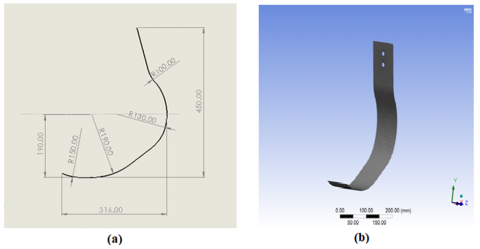Figure 6. Athlete's artificial foot

5. Results and Discussion

The theoretical property is calculated using the rule of mixtures after the composite material's specifications requirements (density ρcomp, volume fraction Vf, and Poisson's ratio v) have been calculated, as shown in Table 4. It can be seen that there is a difference in elastic properties between the different laminate.

## 7.png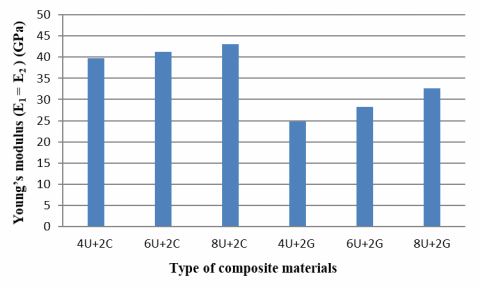Figure 7. Theoretical Young’s modulus of composite materials

Figure 7 shows where an improvement in the mechanical properties appeared when adding layers of woven carbon fibres and woven glass fibres to woven UHMWPE. This improvement was evident in the value of theoretical young’s modulus (E1 = E2) for the composite material that contains carbon fibres due to the fact that young’s modulus carbon fibres have a higher value than glass fibres . The increase in this value indicates the increase in modulus of elasticity of the UHMWPE fibres.

As illustrated in Figure 8, increasing the NO. of UHMWPE layers results in a drop in density due to the increased volume relative to the mass of UHMWPE. As a result, when woven glass fibres are added, the density increases more than when woven carbon fibers are added, resulting in an increase in the model's weight. The reason for this is that glass fibers have a higher density than carbon fibres (Table 1).

By conducting a tensile test according to ASTM No. 638, the experimental young Modulus Eexp and the value of the ultimate tensile stress $\left(\sigma_{x}^{T}\right)_{u l t}$ that the laminate can withstand were calculated, and the results were as shown in Table 5. The results show that the laminate 6 has a higher young Modulus value and tensile stress than the rest of the layers.

As shown in Tables 4 and 5, the longitudinal young modulus is quite close to the theoretical and experimental values. There is a high degree of convergence between the results for the same lamina, as illustrated in Figure 9, and these results correspond with those of Jweeg et al. .

Table 6 shows the numerical value of stress at the x and y directions $\sigma_{\mathrm{x}}, \mathrm{y}$ respectively and the in plane shear stress $\tau_{x y}$ were obtained by used Ansys program when entering isotropic or orthotropic information under the same boundary conditions.

Table 4. The theoretical property of lamination used in the current study

 Property Laminate 1 Laminate 2 Laminate 3 Laminate 4 Laminate 5 Laminate 6 Laminate 7 Laminate 8 Laminate 9 ρcomp (Kg/cm3) 1091.69 1049.4 1035.26 1191.29 1155.83 1096.46 1211.42 1222.33 1220.19 Vf 0.62 0.63 0.65 0.71 0.72 0.70 0.70 0.65 0.70 E1 (GPa) 23.603 27.393 30.759 39.753 41.272 43.116 24.822 28.189 32.639 E2 (GPa) 23.603 27.393 30.759 39.753 41.272 43.116 24.822 28.189 32.639 E3 (GPa) 4.754 5.19 5.681 5.795 6.268 6.38 5.082 5.492 6.294 ν12 0.069 0.065 0.064 0.047 0.05 0.049 0.067 0.065 0.066 ν13 0.5 0.51 0.52 0.46 0.47 0.48 0.47 0.48 0.49 ν23 0.5 0.51 0.52 0.46 0.47 0.48 0.47 0.48 0.49 G12(GPa) 0.68 0.627 0.574 0.549 0.51 0.505 0.621 0.579 0.506 G13(GPa) 1.706 1.988 2.459 2.083 2.353 2.38 1.86 2.178 2.595 G23(GPa) 1.706 1.988 2.459 2.083 2.353 2.38 1.86 2.178 2.595

## 8.png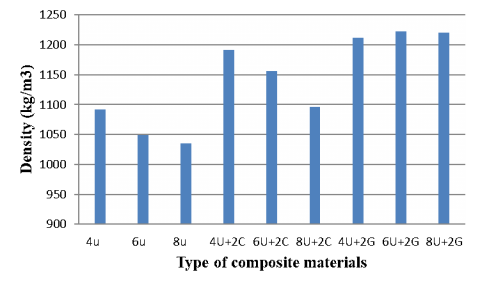Figure 8. Density of composite materials

Table 5. The experimental young modulus of lamination employed in the current study

 Composite lamination ultimate tensile stress (MPa) Experimental longitudinal young’s modulus (GPa) Laminate 1 228 21.768 Laminate 2 255 25.749 Laminate 3 282 28.577 Laminate 4 332 34.962 Laminate 5 376 41.890 Laminate 6 440 43.426 Laminate 7 262 26.165 Laminate 8 282 31.782 Laminate 9 298 34.089

Table 7 shows the result of Tsia–hill for laminate with two types: isotropic and orthotropic. The results show that all laminates have met the conditions of the theory positively. And by observing the values in the table, it becomes clear that there is little difference between the results when using the ISO material or being ortho and with a simple error rate.

As illustrated in Figure 10, the laminate 6 produces the best results. Because it has a lower value than the others, it is regarded as the best on the Tsai-Hill scale. The rationale for this is that the efficiency and resilience to failure of carbon fibres are greater than those of UHMWPE fibres alone or when glass fibres are added to the same number of layers.

## 9.png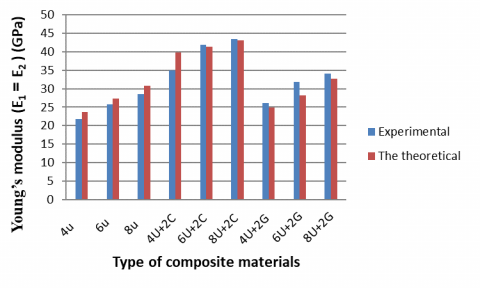Figure 9. Comparison between the theoretical and the experimental young’s modulus

## 10.png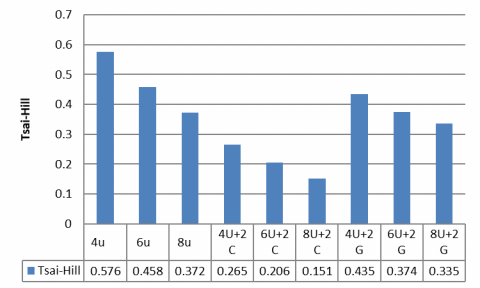Figure 10. Result of Tsai-Hill failure theory

Table 6. The numerical stress at the x and y directions and shear stress

 Composite lamination σx (MPa) σy (MPa) $\tau_{\mathrm{xy}(\mathrm{MPa})}$ Iso Ortho Iso Ortho Iso Ortho Laminate 1 62.6 62.8 145.57 145.1 59.7 59.34 Laminate 2 62.5 62.69 145.3 144.65 59.6 59.18 Laminate 3 62.48 62.61 145.2 144.3 59.6 59 Laminate 4 62.098 62.17 143.87 143.36 59.1 58.68 Laminate 5 62.25 62.14 144.5 143.2 59.3 58.64 Laminate 6 63.28 62.21 144.87 143.36 59.56 58.7 Laminate 7 62.5 62.73 145.4 144.83 59.7 59.24 Laminate 8 62.64 62.67 145.7 144.57 59.84 59.16 Laminate 9 62.7 62.72 144.5 144.55 59.16 59.164

Table 7. Tsai-Hill results

 Composite lamination Tsai-Hill Error % Iso Ortho Laminate 1 0.581 0.576 0. 93 Laminate 2 0.463 0.458 1.16 Laminate 3 0.378 0.372 1.63 Laminate 4 0.268 0.265 1.09 Laminate 5 0.21 0.206 2.02 Laminate 6 0.155 0.151 2.4 Laminate 7 0.44 0.435 1.16 Laminate 8 0.381 0.374 1.91 Laminate 9 0.335 0.335 0.04
6. Conclusions

The study was carried out on a model of a sports prosthetic foot in the J shape, which is used in running competitions. Nine samples were constructed utilizing synthetic fibres (ultrahigh-molecular-weight polyethylene, perlon, carbon fiber, and glass fibre) and the matrix material (epoxy resin orthocryl lamination 80:20 pro), and all essential tests and calculations were performed. For the purpose of selecting the appropriate sample for the manufacture of the sports prosthesis, the following has been remarked:

1. Experimental and theoretical studies indicate that adding carbon fibres to Ultra fibres has a greater effect than adding glass fibres. As the samples formed by adding carbon fibers are stronger and have a lower density. Also, the value of the longitudinal Young’s modulus increased by more than 25% while they have increased and does not exceed 10% when adding fiberglass.

2. Between the experimental and theoretical results, there is a high degree of convergence in the values of the longitudinal Young's modulus.

3. When entering the information of the composite material into the Ansys program in the form of ISO or Ortho, the results of the stresses when analyzing the foot at the same marginal conditions show a great convergence.

4. When applying the theory of failure to examine the manufactured samples, it became clear that the best sample was the sixth sample.

References

 Khalil, Y., Kowalski, A., Hopkinson, N. (2016). Influence of energy density on flexural properties of laser-sintered UHMWPE. Additive Manufacturing, 10: 67-75. https://doi.org/10.1016/j.addma.2016.03.002

 Gay, D., Hoa, S.V. (2007). Composite Materials: Design and Applications. CRC press.

 Bragaru, M., Dekker, R., Geertzen, J.H., Dijkstra, P.U. (2011). Amputees and sports: A systematic review. Sports Medicine, 41(9): 721-740. https://doi.org/10.2165/11590420-000000000-00000

 Hafner, B.J. (2005). Clinical prescription and use of prosthetic foot and ankle mechanisms: A review of the literature. JPO: Journal of Prosthetics and Orthotics, 17(4): S5-S11. https://doi.org/10.1097/00008526-200510001-00004

 Gailey, R. (2005). Functional value of prosthetic foot/ankle systems to the amputee. JPO: Journal of Prosthetics and Orthotics, 17(4): S39-S41. https://doi.org/10.1097/00008526-200510001-00014

 Nolan, L. (2008). Carbon fibre prostheses and running in amputees: A review. Foot and Ankle Surgery, 14(3): 125-129. https://doi.org/10.1016/j.fas.2008.05.007

 Rahman, M., Bennett, T., Glisson, D., Beckley, D., Khan, J. (2014). Finite element analysis of prosthetic running blades using different composite materials to optimize performance. In ASME International Mechanical Engineering Congress and Exposition, 46590: V010T13A013. https://doi.org/10.1115/IMECE2014-37293

 Shasmin, H.N., Osman, N.A., Latif, L.A. (2008). Comparison between biomechanical characteristics of stainless steel and bamboo pylons: A preliminary study. IFMBE Proceedings, 21(1). https://doi.org/10.1007/978-3-540-69139-6-210

 LeMoyne, R. (2016). Passive transtibial prosthesis and associated prosthetic components. In Advances for Prosthetic Technology, pp. 59-68.

 Nielsen, D.H., Shurr, D.G., Golden, J.C., Meier, K. (1988). Comparison of energy cost and gait efficiency during ambulation in below-knee amputees using different prosthetic feet—a preliminary report. JPO: Journal of Prosthetics and Orthotics, 1(1): 24-31.

 Patarata, V.S. (2017). Structural Simulation of 3D Limb Prostheses Using Meshless Methods.

 Sun, J., Voglewede, P.A. (2011). Controller implementation of a powered transtibial prosthetic device. In International Design Engineering Technical Conferences and Computers and Information in Engineering Conference, 54839: 597-603.

 Kumar, P.K., Charan, M., Kanagaraj, S. (2017). Trends and challenges in lower limb prosthesis. IEEE Potentials, 36(1): 19-23. https://doi.org/10.1109/MPOT.2016.2614756

 Hawkins, J. (2015). The Dynamic characterisation of ESR prosthetic running feet: an investigation of the key parameters affecting their performance. Doctoral Dissertation, Bournemouth University.

 Rigney, S.M., Simmons, A., Kark, L. (2017). Mechanical characterization and comparison of energy storage and return prostheses. Medical Engineering & Physics, 41: 90-96. 10.1016/j.medengphy.2017.01.003

 Bhagavathiyappan, S., Balamurugan, M., Rajamanickam, M., Vijayanandh, R., Kumar, G.R., Kumar, M.S. (2020). Comparative computational impact analysis of multi-layer composite materials. In AIP Conference Proceedings, 2270(1): 040007. https://doi.org/10.1063/5.0019380

 Alizadeh, Y. (2020). Design and structural analysis of composite prosthetic running blades for athletes. https://www.researchgate.net/figure/Design-and-dimensions-of-my-J-blade-inspired-by-Cheetah-Xtend-model-by-Ossur_fig1_342336561, accessed on Oct. 5, 2021.

 Jones, R.M. (2018). Mechanics of Composite Materials. CRC Press.

 Callister, W.D., Rethwisch, D.G. (2018). Materials Science and Engineering: An Introduction. New York: Wiley.

 ASTM International. (2014). Standard test method for tensile properties of plastics. Astm International.

 Hyer, M.W., White, S.R. (2009). Stress Analysis of Fiber-Reinforced Composite Materials. DEStech Publications, Inc.

 Kim, Y., Choi, C., Kumar, S.K.S., Kim, C.G., Kim, S.W., Lim, J.H. (2017). Thermo-gravimetric analysis method to determine the fiber volume fraction for PAN-based CFRP considering oxidation of carbon fiber and matrix. Composites Part A: Applied Science and Manufacturing, 102: 40-47. https://doi.org/10.1016/j.compositesa.2017.07.024

 Aly, M.F., Goda, I.G.M., Hassan, G.A. (2010). Experimental investigation of the dynamic characteristics of laminated composite beams. International Journal of Mechanical and Mechatronics, 10(3): 59-68.

 Kumar, G.R., Vijayanandh, R., Kumar, M.S., Kumar, S.S. (2018). Experimental testing and numerical simulation on natural composite for aerospace applications. In AIP Conference Proceedings, 1953(1): 090045. 10.1063/1.5032892

 Bonacini, D. (2011). Method for positioning a bracket-fixable running foot for lower limb prosthesis. European Patent Office 2011.

 Walke, K.M., Pandure, P.S. (2017). Mechanical properties of materials used for prosthetic foot: A review. IOSR Journal of Mechanical and Civil Engineering (IOSR-JMCE), 61: 65. 10.9790/1684-17010026165

 Jweeg, M.J., Hammood, A.S., Al-Waily, M. (2012). Experimental and theoretical studies of mechanical properties for reinforcement fiber types of composite materials. International Journal of Mechanical & Mechatronics Engineering IJMME-IJENS, 12(4): 62-75.